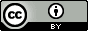## Sunday, September 16, 2012

### The free energy is lowest at equilibrium

This is Figure 10.1 from Dill and Bromberg's Molecular Driving Forces, which is my favorite book on statistical mechanics. It is a beautiful example from a beautiful book.

A system is at equilibrium when its free energy is a minimum
$$dA=0$$ Here I use the Helmholtz free energy $$A=U-TS$$ but the same is true for the Gibbs free energy. Let's explore this for the simple four-bead polymer shown in the figure above. Since I have already defined the probabilities of the folded ($p_f$) and unfolded ($p_{uf}$) macrostates in a previous post it is easiest to start with the expression for the internal energy ($U$) and entropy ($S$) in terms if probabilities. $$U=N\left<\varepsilon\right>=N\sum_i^{\begin{array}{ c }\text{micro} \\ \text{states} \\ \end{array}} \varepsilon_ip_i$$ $$S=-Nk\left<ln(p)\right>=-Nk\sum_i^{\begin{array}{ c }\text{micro} \\ \text{states} \\ \end{array}} p_i\ln(p_i)$$This results in $$U=N\varepsilon_0 p_{uf}\text{ and }S=-Nk\left( p_f\ln(p_f)+p_{uf}\ln\left(\frac{p_{uf}}{4}\right) \right)$$Combining these two terms and using the fact that probabilities must sum to 1 ($p_f+p_{uf}=1$), the free energy can be written as $$A=N\varepsilon_0 (1-p_{f})+TNk\left( p_f\ln(p_f)+(1-p_{f})\ln\left(\frac{(1-p_{f})}{4}\right) \right)$$This allows me to plot $A$ as a function of $p_f$, using $T$ = 298.15 K, $N=N_A$, and $N_A\varepsilon_0=2.5$ kJ/mol
As you can see, the free energy is a minimum when the probability of the folded state is about 0.4  or 0.407 to be more precise - precisely the value predicted by the Boltzmann distribution: $p_f=1/q$.

The total entropy is a maximum at equilibrium
This figure shows the internal energy and entropy contributions to the free energy as function on $p_f$.

When seeing this plot for the first time many people are surprised that the entropy is not a maximum (i.e. $-TS$ is not a minimum) at equilibrium.  Does this simple system violate the second law of thermodynamics? No! I am plotting the entropy of the system and not the total entropy that the second law refers to.  The total entropy is indeed a maximum at equilibrium:$$dA_{\text{system}}=0\\dU_{\text{system}}-TdS_{\text{system}}=0\\-\frac{dU_{\text{system}}}{T}+dS_{\text{system}}=0\\ \frac{dq_{\text{surroundings}}}{T}+dS_{\text{system}}=0\\ dS_{\text{surroundings}}+dS_{\text{system}}=0\\dS_{\text{total}}=0$$ Increasing the number of molecules in the unfolded state requires energy ($U$ increases at $p_f$ decreases) and this energy has to come from somewhere.  It is transferred to the system from the surroundings at heat: $$dq_{\text{surroundings}}=-dU_{\text{system}}$$and this lowers the entropy of the surroundings by$$dS_{\text{surroundings}}=\frac{dq_{\text{surroundings}}}{T}$$The entropy of the system is highest ($-TS$ is lowest) when $p_f=\frac{1}{5}$, i.e. when all microstates are equally populated because this leads to the highest multiplicity ($W_{\text{system}}$):$$S_{\text{system}}=k\ln\left(\frac{N!}{N_f!N_{uf1}!N_{uf2}!N_{uf3}!N_{uf4}!}\right)$$

Some technical stuff you can skip if you want
Another reason you might think $S_{\text{system}}$ should be a maximum at equilibrium is that  the Boltzmann distribution is derived by maximizing the entropy:$$\frac{\partial S}{\partial N_i}=0\text{ for }i=1,2,3.,..$$However, what is actually maximized is a Lagrangian function: $$L=S+\alpha \left( N-\sum_i^{\begin{array}{ c }\text{micro} \\ \text{states} \\ \end{array}}N_i \right)-\beta \left( U-\sum_i^{\begin{array}{ c }\text{micro} \\ \text{states} \\ \end{array}}\varepsilon_i N_i \right)$$that conserves the number of particles ($N$) and the internal energy ($U$).  Because of the latter requirement $$\frac{\partial L}{\partial N_i}=0\text{ for }i=1,2,3.,..$$ maximizes $S_{\text{total}}$ rather than $S_{\text{system}}$ because it is only the total internal energy that is conserved: $$dU_{\text{system}}+dU_{\text{surroundings}}=0$$This work (except the first figure which is © by Garland Science) is licensed under a Creative Commons Attribution 3.0 Unported License.